Next: Uncertainty due to systematic Up: Counting experiments Previous: Binomially distributed observables   Contents

## Poisson distributed quantities

As is well known, the typical application of the Poisson distribution is in counting experiments such as source activity, cross-sections, etc. The unknown parameter to be inferred is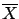. Applying the Bayes formula we get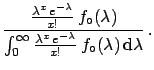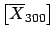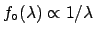(5.53)

Assuming5.7constant up to a certain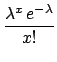and making the integral by parts we obtain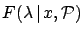(5.54)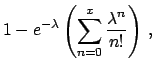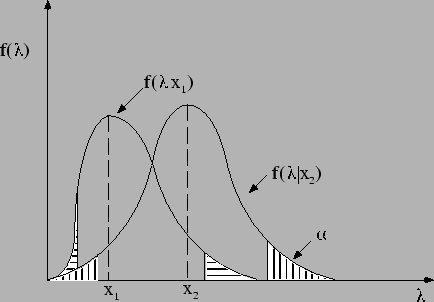(5.55)

where the last result has been obtained by integrating () also by parts. Figureshows how to build the credibility intervals, given a certain measured number of counts.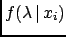Figureshows some numerical examples.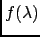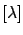has the following properties.

• The expectation values, variance, and value of maximum probability are
 E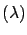(5.56) Var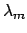(5.57)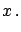(5.58)

The fact that the best estimate ofin the Bayesian sense is not the intuitive valuebutshould neither surprise, nor disappoint us. According to the initial distribution used there are always more possible values ofon the right side than on the left side of'', and they pull the distribution to their side; the full information is always given byand the use of the mean is just a rough approximation; the difference from the desired'' intuitive valuein units of the standard deviation goes as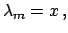and becomes immediately negligible.
• Whenbecomes large we get
 E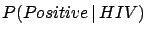(5.59) Var(5.60)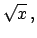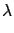(5.61)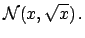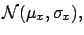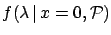(5.62)

() is one of the most familar formulae used by physicists to assess the uncertainty of a measurement, although it is sometimes misused.
Let us conclude with a special case: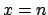. As one might imagine, the inference is highly sensitive to the initial distribution. Let us assume that the experiment was planned with the hope of observing something, i.e. that it could detect a handful of events within its lifetime. With this hypothesis one may use any vague prior function not strongly peaked at zero. We have already come across a similar case in Section, concerning the upper limit of the neutrino mass. There it was shown that reasonable hypotheses based on the positive attitude of the experimentalist are almost equivalent and that they give results consistent with detector performances. Let us use then the uniform distribution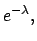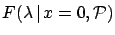(5.63)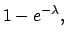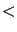(5.64)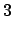at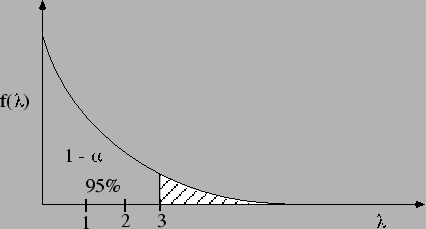probability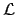(5.65)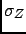Next: Uncertainty due to systematic Up: Counting experiments Previous: Binomially distributed observables   Contents
Giulio D'Agostini 2003-05-15